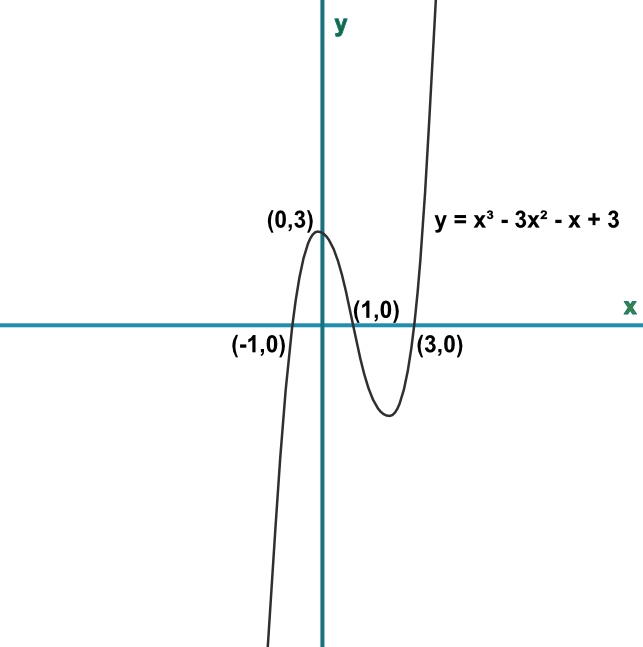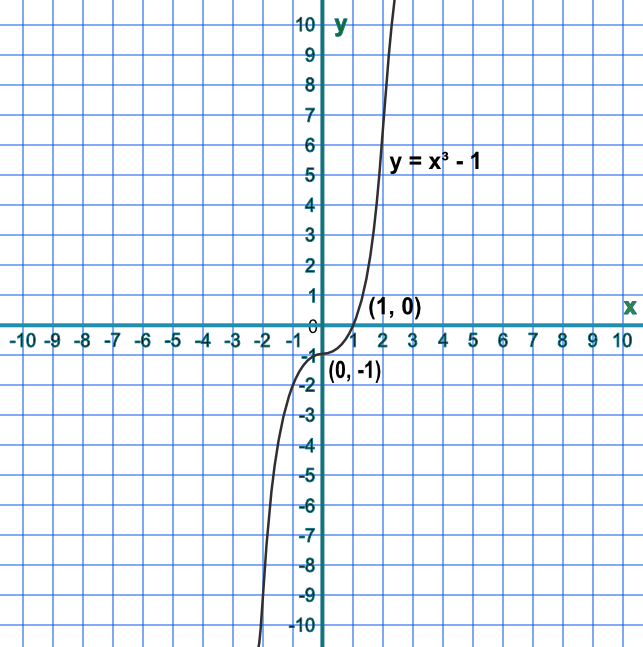Graphing Cubic Functions

## Graphing Cubic Functions

A cubic function will have an x^3 term. An example of a cubic function is 2x^3 + 8x^2 - 2x - 8.

A cubic function will have either one, two or three distinct solutions, where the function crosses the x-axis. This can be determined by factorising the function: the above example factorises to (x + 4)(2x - 2)(x + 1), giving roots of -4, 1 and -1. The function y = (x - 4)^3 has one distinct solution (+4).

The intersection with the y-axis can be obtained by identifying the number part of the function.

## Example 1

Sketch the graph of y = x^3-3x^2-x+3. Mark the points of intersection with both the x and y-axis.

Hint: the function factorises to (x-3)(x-1)(x+1).

Substitute a value into each bracket to make that bracket zero eg for the first bracket, set x=3 to make (x-3)=0 therefore x is a solution. Similarly for the other two brackets.Answer: The roots of the equation are 3, 1 and -1.

## Example 2

How many distinct solutions does y = x^3 - 1 have?

The only solution to x^3-1 is when x=1